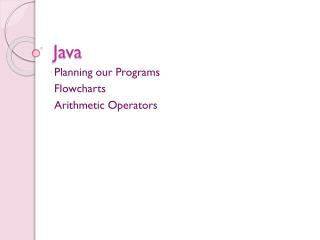# Java - PowerPoint PPT PresentationDownload PresentationJava

JavaDownload Presentation## Java

- - - - - - - - - - - - - - - - - - - - - - - - - - - E N D - - - - - - - - - - - - - - - - - - - - - - - - - - -
##### Presentation Transcript

1. Java Planning our Programs Flowcharts Arithmetic Operators

2. Planning Programs • It is very important to plan our programs before we start coding • There are two ways of planning a program; • Pseudo-code = makes use of English statements to plot the program • Flowcharts = use graphical symbols

3. Example • Lets say we had the following program class VariablesExample { public static void main (String args[]){ //variables are declared and assigned int N1 = 50; int N2 = 13; int tot; //the total of variables N1 and N2 //is stored in tot tot = N1 + N2; //Finally, we can show the result System.out.println(tot); } }

4. Pseudo-code Plan • The following is the Pseudo-code Plan for the previous program; 1. Start 2. Store 50 in N1. 3. Store 13 in N2. 4. Add N1 to N2 and store result in tot. 5. Display the value in tot. 6. Stop

5. Start N1 = 50 N2 = 13 tot = N1 + N2 Display tot End Flowchart Plan • The following is the Flowchart Plan of the previous program;

6. Why do we Plan Programs? • We plan our programs in order to know what we will be doing before we start coding • With a plan it will be much easier to know what structure our program will have • Planning makes programming much easier

7. Flowcharts • A flowchart is basically a graphical presentation of our program • A flowchart is very is to read and understand • Flowcharts break down our programs into many steps

8. Flowchart Symbols

9. Terminator • The terminator is used to show • The start and • The end of a program START END

10. Process • A process is any action to be done by the program • N1 = 50 • N2 = 13 The process in this case is declaring two variables

11. Decision • Decision are used when we have a comparison • Decisions have two outputs which are YES or NO. Is it Raining? YES NO

12. Input/Output • The Input/ Output symbol is used • When the program requires and input • When the program results in an output In this case the output to be shown is the contents of tot • Display tot

13. Try it out … class Variable{ public static void main (String args[]){ //variables are declared and assigned int N1 = 20; int N2 = 10; int tot; int tot2; //tot1 and tot 2 declared tot = N1 + N2; tot2 = N1 – N2; //Finally, we can show the result System.out.println(tot2); } } • Create • The pseudo-codes plan • The flowchart plan for the following program;

14. Arithmetic Operators • Arithmetic Operators are used to perform mathematical calculations • The basic arithmetic operators ; • + (addition) • - (subtraction) • / (division) • * (multiplication) • % (remainder) These are called binary operators because they need to use at least two variables

15. Unary Operators • Then are also what we call unary operators • These only need one variable • ++ (increment by 1) • -- (decrement by 1) • variable += x (same as variable = variable+ x) • variable -= x (same as variable = variable- x) • variable *= x (same as variable = variable* x) • variable /= x (same as variable = variable/ x) • variable %= x (same as variable = variable% x)

16. Combining Operators • We could create a formula by combining a number of operators • The order the operators are work out is the following; • Multiplication • Division • Remainder • Addition • Subtraction X = 10 + 4 * 3 / 2; 10 + 4 * 3 / 2 10 + 12 / 2 10 + 6 16

17. Use of Brackets • When we use brackets in our formula we are telling the program which operations to calculate first X = (10 + 4) * 3 / 2; (10 + 4 ) * 3 / 2 14 * 3 / 2 42 / 2 21 As we can see the result has changed!!